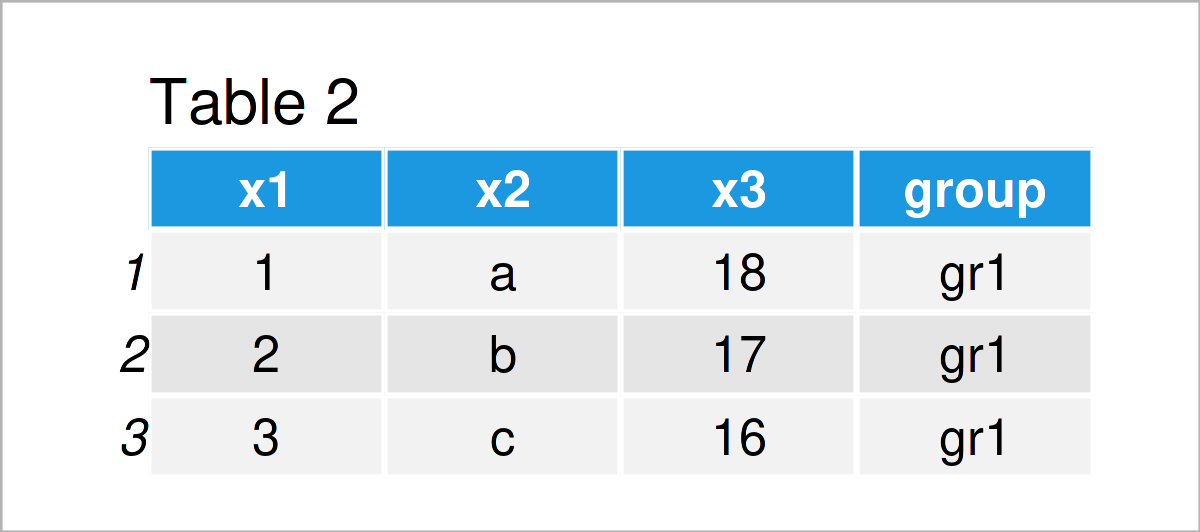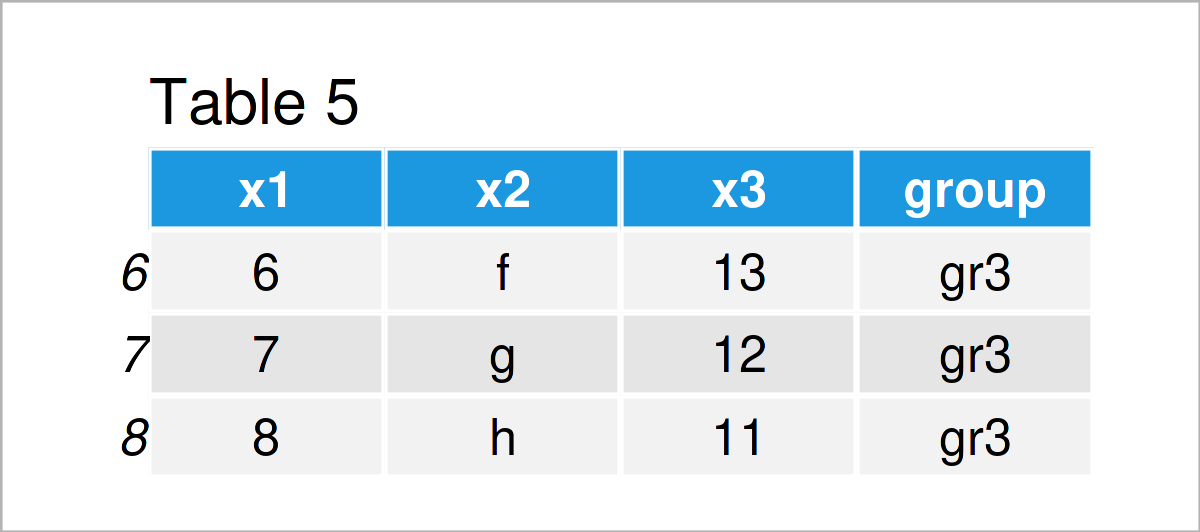# Subset Data Frame within for-Loop in R (Example)

In this tutorial, I’ll show how to split a data frame using a loop in R.

The article will consist of these contents:

So let’s start right away:

## Creation of Example Data

We’ll use the following data as a basis for this R programming tutorial:

```data <- data.frame(x1 = 1:8,            # Create example data frame
x2 = letters[1:8],
x3 = 18:11,
group = c("gr1", "gr1", "gr1",
"gr2", "gr2",
"gr3", "gr3", "gr3"))
data                                    # Print example data frame```Table 1 illustrates that our example data is made up of eight rows and four columns. Note that the fourth column is a group indicator.

## Example: Divide Data Frame Based on Group Column Using split() Function

In this example, I’ll show how to create new data frame subsets within a for-loop.

For this task, we first need to apply the split function to our data frame to create a list of data frame subsets:

```my_splits <- split(data, data\$group)    # Split data frame in list
my_splits                               # Print list
# \$gr1
#   x1 x2 x3 group
# 1  1  a 18   gr1
# 2  2  b 17   gr1
# 3  3  c 16   gr1
#
# \$gr2
#   x1 x2 x3 group
# 4  4  d 15   gr2
# 5  5  e 14   gr2
#
# \$gr3
#   x1 x2 x3 group
# 6  6  f 13   gr3
# 7  7  g 12   gr3
# 8  8  h 11   gr3```

We could now simply extract each of the data frame subsets from this list. For instance, the following R code returns the first data frame subset:

`my_splits\$gr1                           # Print data frame in first list element`However, we can also use a for-loop to create new data frames where each new data frame contains one of these subsets.

For this, we first have to specify a vector of names for our new data frames. Note that this vector needs to have the same length as the number of splits.

```split_names <- c("data_new1",           # Specify names of new data frames
"data_new2",
"data_new3")```

Next, we can run a for-loop where we select one of the data frame subsets within each iteration:

```for (i in 1:length(my_splits)) {        # Run for-loop

assign(split_names[i], my_splits[[i]])
}```

After running the previous R code, we have created three new data frames, where each data frame contains one of the subsets:

`data_new1                               # Print first data frame``data_new2                               # Print second data frame``data_new3                               # Print third data frame`## Video & Further Resources

Do you want to know more about the splitting of a data frame using a loop? Then I recommend watching the following video on my YouTube channel. In the video, I explain the content of this tutorial in RStudio:

Please accept YouTube cookies to play this video. By accepting you will be accessing content from YouTube, a service provided by an external third party.If you accept this notice, your choice will be saved and the page will refresh.

Furthermore, you may want to read the related articles on https://www.statisticsglobe.com/.

In summary: This tutorial has shown how to divide a data frame using a for-loop in the R programming language. Please let me know in the comments, in case you have additional questions.

Subscribe to the Statistics Globe Newsletter